Example Questions

1 2 3 4 5 7 Next →

Example Question #61 : Statistics

Find the mean of the following set of numbers.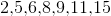Explanation:

To find mean, simply total the numbers and divide by 7.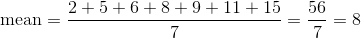Example Question #62 : Statistics

Find the mean of the following set of numbers: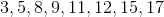Explanation:

To find the mean, simply sum all of the numbers and divide by how many there are.

Thus,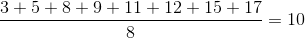Example Question #63 : Statistics

Find the mean of the following set of numbers: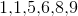Explanation:

To find the mean, simply sum up the numbers and divide by the amount there are. Thus,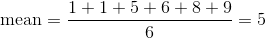Example Question #64 : Statistics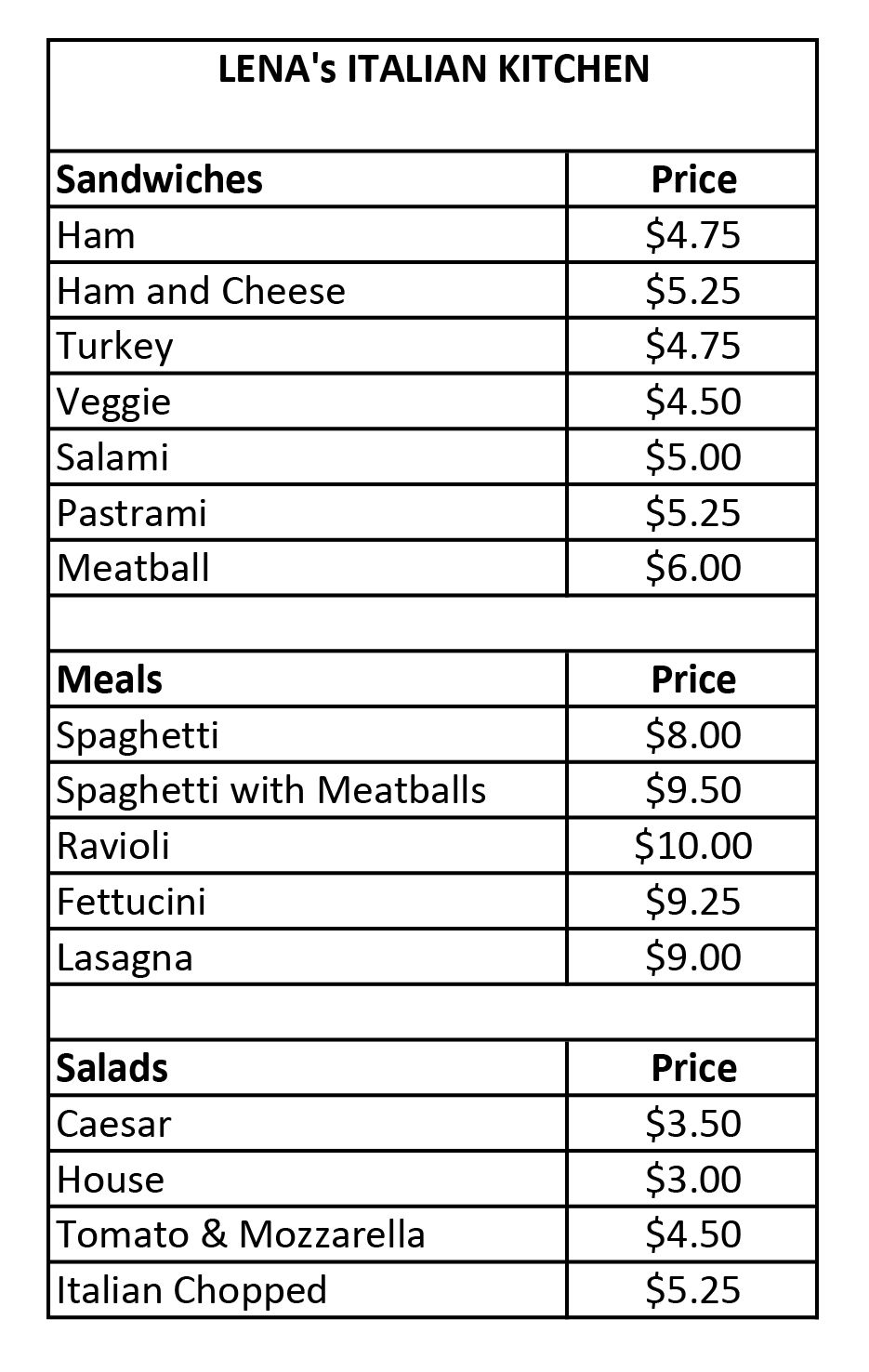From the menu above, what is the average price of a sandwich?  Round to the nearest cent.

$5.07$4.99

$5.11$5.03

$5.09 Correct answer:$5.07

Explanation:

Average  = Sum of the Options / # of Options

= (4.75 + 5.25 + 4.75 + 4.50 + 5.00 +5.25 + 6.00) / 7 = 35.50 / 7 = 5.07

1 2 3 4 5 7 Next →